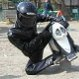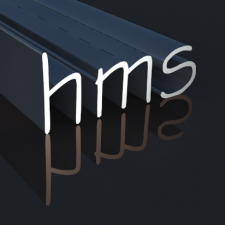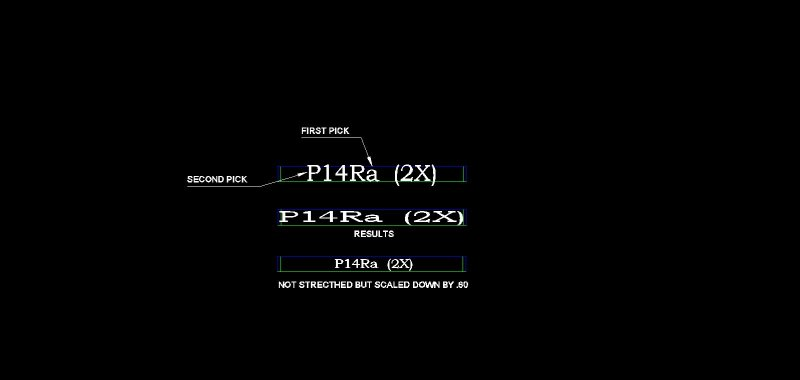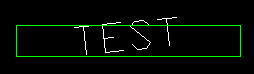Text fit lispRecommended PostsHi All,

I was wondering if there was a lisp routine out there that when you drop a dtext string into a rectanlge that it would shrink down to fit inside? I looked at the text fit command in the express tools but it only shrinks or stretches the length.

Thanks,

Brian

• Replies 22
• Created

Top Posters In This Topic

•5

•3

•3

•9

Top Posters In This Topic

•Lee Mac 5 posts

•Jef! 3 posts

•BrianTFC 3 posts

•vanowm 9 posts

Posted ImagesOne of the text properties is width factor so for a rectang set text height to rectang height width factor would probably need a fudgy factor as III is different length to AAA so depends on actual text content this is known as Kerning. I would do something like a + or - or =accept repeated to get effect. Maybe search for Kern text

found this using MTEXT and an alternate text editor the \T modifier with expand (or contract) the space between letters.

Share on other sitesBrian,

the express tools command, text fit, uses the width factor to shrinks or stretches the text string to match the desired length, if I understand correctly, you also want a match in height.

Perhaps something like this

(defun c:test (/      ss     esel   txtobj old_osm  ll ur
ll1    ur1    llpt   urpt   ulpt   brpt  llpt1 urpt1
ulpt1  brpt1  scf    nscf
)
(prompt "\n Select the rectangle to fit text inside: ")
(if
(and (setq ss (ssget "_:S:E" '((0 . "LWPOLYLINE"))))
(setq esel (entsel "\nSelect the text to fit in the rectangle: "))
)
(progn
(setq txtobj (vlax-ename->vla-object (car esel)))
(if (equal (vla-get-ObjectName txtobj) "AcDbText")
(progn
(setq old_osm (getvar "osmode"))
(setvar "osmode" 0)
(vla-getboundingbox
(vlax-ename->vla-object (ssname ss 0))
'll
'ur
)
(vla-getboundingbox txtobj 'll1 'ur1)
(setq llpt  (trans (vlax-safearray->list ll) 0 1)
urpt  (trans (vlax-safearray->list ur) 0 1)
llpt1 (trans (vlax-safearray->list ll1) 0 1)
)
;; setq
(vl-cmdf "move" (car esel) "" llpt1 llpt)
(vla-update txtobj)
(vla-getboundingbox txtobj 'll1 'ur1)
(setq llpt1 (trans (vlax-safearray->list ll1) 0 1)
urpt1 (trans (vlax-safearray->list ur1) 0 1)
)
;; setq
(vl-cmdf "scale" (car esel) "" llpt "R" "@" ulpt1 ulpt "")
(vla-update txtobj)
(vla-getboundingbox txtobj 'll1 'ur1)
(setq llpt1 (trans (vlax-safearray->list ll1) 0 1)
urpt1 (trans (vlax-safearray->list ur1) 0 1)
scf   (vla-get-scalefactor txtobj)
nscf  (/ (* (distance llpt brpt) scf) (distance llpt1 brpt1))
)
;; setq
(vla-put-scalefactor txtobj nscf)
(setvar "osmode" old_osm)
)
;; progn
)
;; if
)
;; progn
)
;; if
)

Henrique

Share on other sitesHenrique,

I was wondering if there is way for the text to be scaled down to fit inside vs. stretched and shrinked to fit?

Thanks,

BrianTEXT SHRINK.dwg

Share on other sitesTry this quickly written code:

([color=BLUE]defun[/color] c:tfit ( [color=BLUE]/[/color] ln pl pt tx )
([color=BLUE]if[/color]
([color=BLUE]and[/color]
([color=BLUE]setq[/color] pl (LM:selectifobject [color=MAROON]"\nSelect LWPolyline: "[/color] [color=MAROON]"LWPOLYLINE"[/color]))
([color=BLUE]setq[/color] tx (LM:selectifobject [color=MAROON]"\nSelect Text: "[/color] [color=MAROON]"TEXT"[/color]))
([color=BLUE]setq[/color] pl ([color=BLUE]mapcar[/color] '[color=BLUE]cdr[/color] ([color=BLUE]vl-remove-if-not[/color] '([color=BLUE]lambda[/color] ( x ) ([color=BLUE]=[/color] 10 ([color=BLUE]car[/color] x))) ([color=BLUE]entget[/color] pl)))
ln ([color=BLUE]length[/color] pl)
pt ([color=BLUE]mapcar[/color] '[color=BLUE]/[/color] ([color=BLUE]apply[/color] '[color=BLUE]mapcar[/color] ([color=BLUE]cons[/color] '[color=BLUE]+[/color] pl)) ([color=BLUE]list[/color] ln ln))
tx ([color=BLUE]entget[/color] tx)
)
)
([color=BLUE]entmod[/color]
([color=BLUE]subst[/color] '(72 . 1) ([color=BLUE]assoc[/color] 72 tx)
([color=BLUE]subst[/color] '(73 . 2) ([color=BLUE]assoc[/color] 73 tx)
([color=BLUE]subst[/color] ([color=BLUE]cons[/color] 10 pt) ([color=BLUE]assoc[/color] 10 tx)
([color=BLUE]subst[/color] ([color=BLUE]cons[/color] 11 pt) ([color=BLUE]assoc[/color] 11 tx)
([color=BLUE]subst[/color]
([color=BLUE]cons[/color] 40
([color=BLUE]*[/color] [highlight]0.8 [color=GREEN];; Text Gap[/highlight][/color]
([color=BLUE]-[/color]
([color=BLUE]cadr[/color] ([color=BLUE]apply[/color] '[color=BLUE]mapcar[/color] ([color=BLUE]cons[/color] '[color=BLUE]max[/color] pl)))
([color=BLUE]cadr[/color] ([color=BLUE]apply[/color] '[color=BLUE]mapcar[/color] ([color=BLUE]cons[/color] '[color=BLUE]min[/color] pl)))
)
)
)
([color=BLUE]assoc[/color] 40 tx)
tx
)
)
)
)
)
)
)
([color=BLUE]princ[/color])
)

[color=GREEN];; Select if Object  -  Lee Mac[/color]
[color=GREEN];; Continuously prompts the user for a selection of a specific object[/color]

([color=BLUE]defun[/color] LM:SelectifObject ( msg obj [color=BLUE]/[/color] ent )
([color=BLUE]while[/color]
([color=BLUE]progn[/color] ([color=BLUE]setvar[/color] 'errno 0) ([color=BLUE]setq[/color] ent ([color=BLUE]car[/color] ([color=BLUE]entsel[/color] msg)))
([color=BLUE]cond[/color]
(   ([color=BLUE]=[/color] 7 ([color=BLUE]getvar[/color] 'errno))
([color=BLUE]princ[/color] [color=MAROON]"\nMissed, try again."[/color])
)
(   ([color=BLUE]=[/color] 'ename ([color=BLUE]type[/color] ent))
([color=BLUE]if[/color] ([color=BLUE]/=[/color] obj ([color=BLUE]cdr[/color] ([color=BLUE]assoc[/color] 0 ([color=BLUE]entget[/color] ent))))
([color=BLUE]princ[/color] [color=MAROON]"\nInvalid Object Selected."[/color])
)
)
)
)
)
ent
)
([color=BLUE]princ[/color])

Adjust the highlighted text gap if necessary.

Share on other sitesLee,

That's exactly what i was looking for, after looking at your code i found the line that if i need to make the text smaller i can. I really do appreciate all the help i get from all of you on on CadForum. Slowly but surely i'm learning how to write small simple routines but i'm getting better at reading the codes and leaning what each line does through Visual Lisp editor, Henrique aka (hmsilvia) showed me how to animate the lisp routine so i can follow how it works.

Thanks again guys,

Brian

Share on other sitesYou're welcome Brian - and of course, ask if you have any questions about the code.

Share on other sites

• 2 years later...Hello.

Thanks for the code, Lee Mac, it works, but not in every situations. for example:Share on other sitesThe following may perform better:

([color=BLUE]defun[/color] c:tfit ( [color=BLUE]/[/color] ln pl pt tx )
([color=BLUE]if[/color]
([color=BLUE]and[/color]
([color=BLUE]setq[/color] tx (LM:selectifobject [color=MAROON]"\nSelect text: "[/color] [color=MAROON]"TEXT"[/color]))
([color=BLUE]setq[/color] pl (LM:selectifobject [color=MAROON]"\nSelect polyline: "[/color] [color=MAROON]"LWPOLYLINE"[/color]))
([color=BLUE]setq[/color] pl ([color=BLUE]mapcar[/color] '[color=BLUE]cdr[/color] ([color=BLUE]vl-remove-if-not[/color] '([color=BLUE]lambda[/color] ( x ) ([color=BLUE]=[/color] 10 ([color=BLUE]car[/color] x))) ([color=BLUE]entget[/color] pl)))
pl ([color=BLUE]mapcar[/color] '([color=BLUE]lambda[/color] ( x ) ([color=BLUE]apply[/color] '[color=BLUE]mapcar[/color] ([color=BLUE]cons[/color] x pl))) '([color=BLUE]min[/color] [color=BLUE]max[/color]))
tx ([color=BLUE]entget[/color]  tx)
tb ([color=BLUE]textbox[/color] tx)
vc ([color=BLUE]mapcar[/color] '[color=BLUE]-[/color] (avgpt pl) ([color=BLUE]mapcar[/color] '[color=BLUE]+[/color] ([color=BLUE]cdr[/color] ([color=BLUE]assoc[/color] 10 tx)) (avgpt tb)))
)
)
([color=BLUE]entmod[/color]
([color=BLUE]subst[/color] '(72 . 1) ([color=BLUE]assoc[/color] 72 tx)
([color=BLUE]subst[/color] '(73 . 2) ([color=BLUE]assoc[/color] 73 tx)
([color=BLUE]subst[/color] ([color=BLUE]cons[/color] 11 ([color=BLUE]mapcar[/color] '[color=BLUE]+[/color] ([color=BLUE]cdr[/color] ([color=BLUE]assoc[/color] 11 tx)) vc)) ([color=BLUE]assoc[/color] 11 tx)
([color=BLUE]subst[/color]
([color=BLUE]cons[/color] 40
([color=BLUE]*[/color]  ([color=BLUE]cdr[/color] ([color=BLUE]assoc[/color] 40 tx)) [highlight]0.9 [color=green];; Alter this to suit[/color][/highlight]
([color=BLUE]apply[/color] '[color=BLUE]min[/color]
([color=BLUE]mapcar[/color] '[color=BLUE]/[/color]
([color=BLUE]apply[/color] '[color=BLUE]mapcar[/color] ([color=BLUE]cons[/color] '[color=BLUE]-[/color] ([color=BLUE]reverse[/color] pl)))
([color=BLUE]apply[/color] '[color=BLUE]mapcar[/color] ([color=BLUE]cons[/color] '[color=BLUE]-[/color] ([color=BLUE]reverse[/color] ([color=BLUE]textbox[/color] tx))))
)
)
)
)
([color=BLUE]assoc[/color] 40 tx) tx
)
)
)
)
)
)
([color=BLUE]princ[/color])
)

([color=BLUE]defun[/color] avgpt ( lst )
(([color=BLUE]lambda[/color] ( len ) ([color=BLUE]mapcar[/color] '[color=BLUE]/[/color] ([color=BLUE]apply[/color] '[color=BLUE]mapcar[/color] ([color=BLUE]cons[/color] '[color=BLUE]+[/color] lst)) ([color=BLUE]list[/color] len len))) ([color=BLUE]length[/color] lst))
)

[color=GREEN];; Select if Object  -  Lee Mac[/color]
[color=GREEN];; Continuously prompts the user for a selection of a specific object[/color]

([color=BLUE]defun[/color] LM:selectifobject ( msg obj [color=BLUE]/[/color] ent )
([color=BLUE]while[/color]
([color=BLUE]progn[/color] ([color=BLUE]setvar[/color] 'errno 0) ([color=BLUE]setq[/color] ent ([color=BLUE]car[/color] ([color=BLUE]entsel[/color] msg)))
([color=BLUE]cond[/color]
(   ([color=BLUE]=[/color] 7 ([color=BLUE]getvar[/color] 'errno))
([color=BLUE]princ[/color] [color=MAROON]"\nMissed, try again."[/color])
)
(   ([color=BLUE]=[/color] 'ename ([color=BLUE]type[/color] ent))
([color=BLUE]if[/color] ([color=BLUE]/=[/color] obj ([color=BLUE]cdr[/color] ([color=BLUE]assoc[/color] 0 ([color=BLUE]entget[/color] ent))))
([color=BLUE]princ[/color] [color=MAROON]"\nInvalid Object Selected."[/color])
)
)
)
)
)
ent
)
([color=BLUE]princ[/color])

Share on other sites

• 2 weeks later...Any luck with the updated program?

Share on other sitesThank you very much. It works great with text that has justify set to middle center. However if justify set to anything else, it moves text outside the box.

Share on other sites(defun c:tfit ( / ln pl pt tx )
(and
(setq tx (LM:selectifobject "\nSelect text: " "TEXT"))
(setq pl (LM:selectifobject "\nSelect polyline: " "LWPOLYLINE"))
(setq pl (mapcar 'cdr (vl-remove-if-not '(lambda ( x ) (= 10 (car x))) (entget pl)))
pl (mapcar '(lambda ( x ) (apply 'mapcar (cons x pl))) '(min max))
tx (entget tx)
)
(entmod
(subst
(cons 40
(*  (cdr (assoc 40 tx)) 0.9 ;; Alter this to suit
(apply 'min
(mapcar '/
(apply 'mapcar (cons '- (reverse pl)))
(apply 'mapcar (cons '- (reverse (textbox tx))))
)
)
)
)
(assoc 40 tx) tx
)
)
(setq tx (entget (cdr (assoc -1 tx)))
tb (textbox tx)
vc (mapcar '- (avgpt pl) (mapcar '+ (cdr (assoc 10 tx)) (avgpt tb)))
)
(entmod
(subst (cons 10 (mapcar '+ (cdr (assoc 10 tx)) vc)) (assoc 10 tx)
(subst (cons 11 (mapcar '+ (cdr (assoc 11 tx)) vc)) (assoc 11 tx) tx)
)
)
)
(princ)
)

(defun avgpt ( lst )
((lambda ( len ) (mapcar '/ (apply 'mapcar (cons '+ lst)) (list len len))) (length lst))
)

;; Select if Object  -  Lee Mac
;; Continuously prompts the user for a selection of a specific object

(defun LM:selectifobject ( msg obj / ent )
(while
(progn (setvar 'errno 0) (setq ent (car (entsel msg)))
(cond
(   (= 7 (getvar 'errno))
(princ "\nMissed, try again.")
)
(   (= 'ename (type ent))
(if (/= obj (cdr (assoc 0 (entget ent))))
(princ "\nInvalid Object Selected.")
)
)
)
)
)
ent
)
(princ)

Share on other sitesThis works perfectly! Thank you very much.

now I'm trying expand this routine to point inside an object instead of selecting it, so it would work with overlapping objects. Command boundary comes to mind:

;        (setq pl2 (LM:selectifobject "\nSelect polyline: " "LWPOLYLINE"))
(command "-boundary" (getpoint) "")
(setq pl (entlast))

however for some reason the script doesn't advance beyond boundary command, it just stops. Any ideas why?

Thank you.

Share on other sitesIt was a face palm right there...all these commands are inside of (and ...) and boundary command returns nil.

This seems to work now:

(defun c:tfit ( / ln pl pt tx )
(SETVAR "cmdecho" 0)
(and
(setq tx (LM:selectifobject "\nSelect text: " "TEXT"))
;        (setq pl (LM:selectifobject "\nSelect polyline: " "LWPOLYLINE"))
(setq pl (getpoint "\nSelect a point: "))
(not (command "-boundary" pl ""))
(setq pl (entlast))
(setq pl (mapcar 'cdr (vl-remove-if-not '(lambda ( x ) (= 10 (car x))) (entget pl)))
pl (mapcar '(lambda ( x ) (apply 'mapcar (cons x pl))) '(min max))
tx (entget tx)
)
(not (command "_.undo" ""))
(entmod
(subst
(cons 40
(*  (cdr (assoc 40 tx)) 0.9 ;; Alter this to suit
(apply 'min
(mapcar '/
(apply 'mapcar (cons '- (reverse pl)))
(apply 'mapcar (cons '- (reverse (textbox tx))))
)
)
)
)
(assoc 40 tx) tx
)
)
(setq tx (entget (cdr (assoc -1 tx)))
tb (textbox tx)
vc (mapcar '- (avgpt pl) (mapcar '+ (cdr (assoc 10 tx)) (avgpt tb)))
)
(entmod
(subst (cons 10 (mapcar '+ (cdr (assoc 10 tx)) vc)) (assoc 10 tx)
(subst (cons 11 (mapcar '+ (cdr (assoc 11 tx)) vc)) (assoc 11 tx) tx)
)
)
)
(SETVAR "cmdecho" 1)
(princ)
)

One issue found though, if text is on an angle, it doesn't fit properlyShare on other sites

• 1 year later...Can anyone help with angled text?

Thank you.

Share on other sitesI think I fixed it, thanks Lee Mac for bounding box routine:

(DEFUN c:tfit (/ ln pl pt tx)
(AND
(SETQ tx (LM:selectifobject "\nSelect text: " "TEXT"))
;        (setq pl (LM:selectifobject "\nSelect polyline: " "LWPOLYLINE"))
(SETQ pl (GETPOINT "\nSelect a point: ")
)
(tfit (ENTGET tx) pl)
)
(PRINC)
)
(DEFUN tfit (tx pl / tb vc x)
(SETVAR "cmdecho" 0)
(AND
tx
pl
(NOT (COMMAND "-boundary" pl ""))
(SETQ pl (ENTLAST))
(SETQ pl (MAPCAR 'CDR (VL-REMOVE-IF-NOT '(LAMBDA (x) (= 10 (CAR x))) (ENTGET pl)))
pl (MAPCAR '(LAMBDA (x) (APPLY 'MAPCAR (CONS x pl))) '(MIN MAX))
)
(NOT (COMMAND "_.undo" ""))
(ENTMOD
(SUBST
(CONS 40
(* (CDR (ASSOC 40 tx))
0.9 ; Alter this to suit
(APPLY 'MIN
(MAPCAR '/
(APPLY 'MAPCAR (CONS '- (REVERSE pl)))
(APPLY 'MAPCAR (CONS '- (REVERSE (_TEXTBOX tx nil))))
)
)
)
)
(ASSOC 40 tx)
tx
)
)

(SETQ tx  (ENTGET (CDR (ASSOC -1 tx)))
tb  (_TEXTBOX tx nil)
vc  (MAPCAR '- (avgpt pl) (MAPCAR '+ (CDR (ASSOC 10 tx)) (avgpt tb)))
add (MAPCAR '- (CDR (ASSOC 10 tx)) (CAR (_textbox tx T)))
)
(ENTMOD
(SUBST (CONS 10 (MAPCAR '+ (CDR (ASSOC 10 tx)) vc add))
(ASSOC 10 tx)
(SUBST (CONS 11 (MAPCAR '+ (CDR (ASSOC 11 tx)) vc add)) (ASSOC 11 tx) tx)
)
)
)
(SETVAR "cmdecho" 1)
(PRINC)
)

(DEFUN avgpt (lst)
(MAPCAR '/ (APPLY 'MAPCAR (CONS '+ lst)) (LIST 2 2))
)

;; Select if Object  -  Lee Mac
;; Continuously prompts the user for a selection of a specific object

(DEFUN LM:selectifobject (msg obj / ent)
(WHILE
(PROGN (SETVAR 'errno 0)
(SETQ ent (CAR (ENTSEL msg)))
(COND
((= 7 (GETVAR 'errno))
(PRINC "\nMissed, try again.")
)
((= 'ename (TYPE ent))
(IF (/= obj (CDR (ASSOC 0 (ENTGET ent))))
(PRINC "\nInvalid Object Selected.")
)
)
)
)
)
ent
)

;; Selection Set Bounding Box  -  Lee Mac
;; Returns a list of the lower-left and upper-right WCS coordinates of a
;; rectangular frame bounding all objects in a supplied selection set.
;; s - [sel] Selection set for which to return bounding box

(DEFUN _textbox (s coords / a b c m n o ll ur)
(SETQ a nil
b nil
m nil
n nil
)
(SETQ o (VLAX-ENAME->VLA-OBJECT (CDR (ASSOC -1 s))))
(IF (AND o
(VLAX-METHOD-APPLICABLE-P o 'getboundingbox)
(NOT (VL-CATCH-ALL-ERROR-P
(VL-CATCH-ALL-APPLY 'VLA-GETBOUNDINGBOX (LIST o 'a 'b))
)
)
)
(SETQ m (CONS (VLAX-SAFEARRAY->LIST a) m)
n (CONS (VLAX-SAFEARRAY->LIST b) n)
)
)
(SETQ c  (MAPCAR '(LAMBDA (a b) (APPLY 'MAPCAR (CONS a b))) '(MIN MAX) (LIST m n))
ll (CAR c)
)
(IF (NOT coords)
(SETQ c (LIST (LIST 0 0 (NTH 2 (CAR c)))
(LIST (ABS (- (CAR ll) (CAR ur)))
)

)
)
)
c
)

Share on other sites

• 1 year later...Hi There

Is it possible to make Lee`s code for mtexts?

Thanks...

Share on other sites

• 4 months later...All of the sudden the last code I posted above stopped working, it now gets error "divide by zero", pointing to

(MAPCAR '/
(APPLY 'MAPCAR (CONS '- (REVERSE pl)))
(APPLY 'MAPCAR (CONS '- (REVERSE (_TEXTBOX tx nil))))
)

Any ideas why?

Share on other sites28 minutes ago, vanowm said:

Any ideas why?

divide by 0 error is (drum roll) because you divide by zero!

adding a little surveillance cam like this

(MAPCAR '/
(setq tmp1 (APPLY 'MAPCAR (CONS '- (REVERSE pl))))
(setq tmp2 (APPLY 'MAPCAR (CONS '- (REVERSE (_TEXTBOX tx nil)))))
)

which after crash help me retrieve tmp1 and tmp2. Here's what is in them

Command: !tmp1
(0.0 0.0 0.0)
Command: !tmp2
(0.729203 0.242907 0.0)

the mapcar divide the 1rst element of tmp1 by  the 1rst element of tmp2 (0.0 / 0.729203)

the mapcar then divide the 2nd element of tmp1 by  the 2nd element of tmp2 (0.0 / 0.242907)

the mapcar then divide the 3rd element of tmp1 by  the 3rd element of tmp2 (0.0 / 0.0). (bombs here...)

I always had that same values in tmp1/tmp2 no matter the position of of the text. After digging out to understand the code, here'S what I found out. The problem is the boundary command. If if fails to create a boundary, entlast will retrieve something else (in my case the text). a pline will have only 2 sets of coords in assoc 10, so tmp1 has 2 elements, so the 3rd element of tmp2, which is 0 is ignored.

ie: (mapcar '/ '(10 10)'(2 2 0)) returns (5 5)

When boundary fails to be created, in my case the text was selected, which has a set of x y z coords. When it happens, here' what is in PL just before you try to mapcar the division

((226.922 97.1085 0.0) (226.922 97.1085 0.0))

What you could do, get/store the entlast before boundary. After boundary, if the "new" entlast is different the one that you stored, proceed, else you know the boundary creation failed, and could prompt a message before quitting.

Share on other sitesIn my case the boundary being created successfully (creates POLYLINE on top of LWPOLYLINE) , it's for some reason coordinates of it in assoc 10 is (0.0 0.0 0.0)

In fact there is no any coordinates available in that boundary polyline:

((-1 . <Entity name: 405fe970>) (0 . "POLYLINE") (330 . <Entity name:
40262cf8>) (5 . "196") (100 . "AcDbEntity") (67 . 0) (410 . "Model") (8 . "0")
(100 . "AcDb2dPolyline") (66 . 1) (10 0.0 0.0 0.0) (70 . 1) (40 . 0.0) (41 .
0.0) (210 0.0 0.0 1.0) (71 . 0) (72 . 0) (73 . 0) (74 . 0) (75 . 0))

This used to work flawlessly before, there must be some setting I've changed that broke it...

Edited by vanowm

Join the conversation

You can post now and register later. If you have an account, sign in now to post with your account.
Note: Your post will require moderator approval before it will be visible.×   Pasted as rich text.   Restore formatting

Only 75 emoji are allowed.